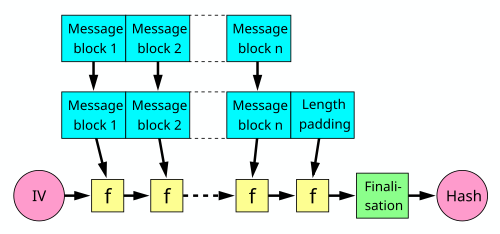## Friday, February 20, 2015

### 52 Things: Number 20: How are Merkle-Damgaard style hash functions constructed?

This is the latest in a series of blog posts to address the list of '52 Things Every PhD Student Should Know' to do Cryptography: a set of questions compiled to give PhD candidates a sense of what they should know by the end of their first year. To finish the basic schemes section, we look at one of the most popular hash function designs...
A Merkle-Damgaard (MD) hash function is a hash function built by extending the domain of a collision-resistant compression function that preserves the collision resistance. This means we can take a small (and fixed) width compression function, prove it is secure and then use it to make a variable length hash function.  Whilst other methods for building hash functions exist, MD is by far the most "popular" (well, most frequently used at least!), with examples including MD5,SHA1 and SHA2. So, time to break those terms down:

#### Secure Hash Function?

Traditionally, a secure hash function $h$ should be:
• Pre-image resistant: given $h(x)$, it is hard to find $x$.
• Second pre-image resistance: given $x$, it is hard to find $y$ such that $h(x)=h(y)$.
• Collision Resistance: It is hard to find $x,y$ such that $h(x)=h(y)$.
If a hash function is collision then clearly it must be second pre-image resistant, so it is this [collision resistance] that we will focus on.

#### Compression Function

A compression function $f:\{0,1\}^n\times\{0,1\}^r\to\{0,1\}^n$ is a function that, as the name suggest, compresses $n+b$-bits worth of input into an $n$-bit output. As you might expect, a collision resistant compression function is a compression function that is collision resistant. So, it can be thought of as a fixed input length hash function, but what happens if we want our hash function to be secure for any input length?

#### The MD hash function construction

The MD construction provides a method for extending the domain of a fixed length compression function into a variable input length hash function. Using a compression function $f$ as above, we are going to use the $n$-bit value as our internal state, and feed in $r$-bits each iteration (it's quite common to set $r=n$). To do this, we begin with an initial value (IV) and split the message $M$ up into blocks of $r$ bits $M=M_0M_1\cdots M_m$, and then simply iterate the construction by setting:
$$S_0:=IV; \qquad i=0,\dots,m-1: S_{i+1}=f(S_i,M_i); \qquad h(M):=S_m$$
Confused? Perhaps the the following diagram will help:Diagram of the MD construction (from Wikipedia)
The most important thing about the MD-construction is that if the compression function is collision resistant, then so is the overall construction (as proven by Merkle). This gives us a secure method for building hash functions out of a smaller, easier studied primitives.

#### Length Extension

You might notice that the diagram has an extra stage that my description didn't: the "finalisation" stage. This is to prevent length extension attacks. For an example, if $N$ is a single block (ie $N\in\{0,1\}^r$) if the attacker knows $h(M)=x$, then he can very easily calculate $h(M||N)$, because $h(M||N)=f(M,N)$. So, some form of finalisation function has to be used to break this relationship.